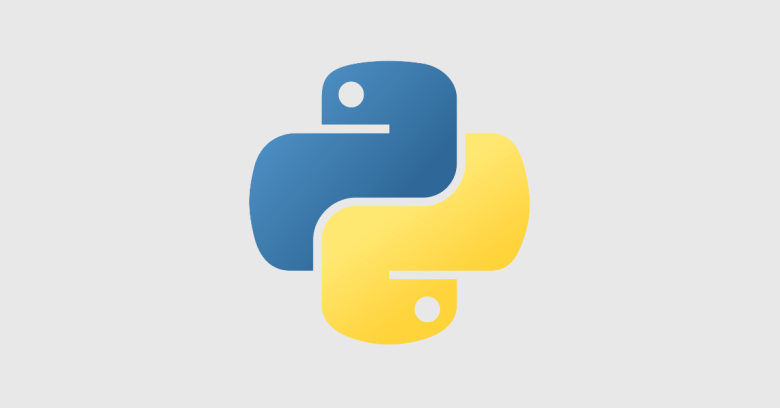# Python: run functions in parallel with a multiprocessing wrapper functionOne common way to run functions in parallel with Python is to use the multiprocessing module which is powerful, it has many options to configure and a lot of things to tweak.

### But what about if we want just a very simple functionality like running a number of functions in parallel and nothing else?

We can use the following wrapper function for this:

``````def run_parallel(*functions):
'''
Run functions in parallel
'''
from multiprocessing import Process
processes = []
for function in functions:
proc = Process(target=function)
proc.start()
processes.append(proc)
for proc in processes:
proc.join()
``````

This function allows us to input as parameter any number of functions with or without their parameters and executed in parallel.

### Example

``````#!/usr/bin/env python3def task_a():
print('Hello: %s'%(msg))def run_parallel(*functions):
'''
Run functions in parallel
'''
from multiprocessing import Process
processes = []
for function in functions:
proc = Process(target=function)
proc.start()
processes.append(proc)
for proc in processes:
proc.join()if __name__ == '__main__':
``````

Executing this will bring the following results:

``````this is task a
Hello: konstantinos
``````

This is nice and simple, but how we will get results back from the functions?

To do this we need to use a shared variable like a list and append the results of the function in the list:

``````#!/usr/bin/env python3
results = []
res = {}
res = {}
res = {}
results.append(res)def run_parallel(*functions):
'''
Run functions in parallel
'''
from multiprocessing import Process
processes = []
for function in functions:
proc = Process(target=function)
proc.start()
processes.append(proc)
for proc in processes:
``````[{'task_a': 2}, {'task_b': 4}, {'task_c': 'Name: konstantinos'}]